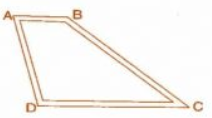Q

# Dinesh went from place A to place B and from there to place C. A is 7.5 km from B and B is 12.7 km from C. Ayub went from place A to place D and from there to place C. D is 9.3 km from A and C is 11.8 km from D. Who travelled more and by how much ?

7. Dinesh went from place A to place B and from  there to place C. A is 7.5 km from B and B is 12.7 km from C.          Ayub went from place A to place D and from there to place C. D is 9.3 km from A and C is 11.8 km from D.                   Who travelled more and by how much?Views

Total distance travelled by Dinesh = AB + BC

=  7.5km + 12.7km

= 20.2 km

Total distance travelled by Ayub = AD + DC

= 9.3km + 11.8 km

=  21.1 km

Hence Ayub travelled More distance than Ayub as 21.1 > 20.2

The difference between path travelled by them = 21.1km - 20.2 km

= 0.9 km.

Hence Ayub travelled 0.9 km more than Dinesh.

Exams
Articles
Questions